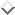Verb SecondNext: Other extensions Up: Problems and Extensions Previous: Delayed evaluation of

### Verb Second

BUG1 can correctly handle analyses that make use of empty elements such as in cases of gap threading analyses of wh-movement, topicalization and relativization . In such cases a possible pivot for, say, a nounphrase can be the empty noun phrase whose semantics obviously unifies with the input; this pivot can easily be connected to the noun phrase goal. As usual the threading of information will check whether the gap is properly related to an antecedent. In section 3.5 I will give some experimental results of a grammar that includes topicalization.

In case the head has been `moved' however there will be a problem for BUG1. Consider the analysis of verb second phenomena in Dutch and German. In most traditional analyses it is assumed that the verb in root sentences has been `moved' from the final position to the second position. Koster  convincingly argues for this analysis of Dutch. Thus a simple root sentence in German and Dutch usually is analysed as in the following examples:In such an analysis can easily be defined, by percolating the information of the verb second position to some empty verb in the final position. Consider the following simple grammar for Dutch:

```node( s/Sem, P0-PN) --->                  % s -> Subj, v, vp
[ node(vp(Np,[],V)/Sem,P2-PN),
node(Np,P0-P1),
node(V,P1-P2)].

node( vp(Subj,T,V)/LF, P0-PN) --->        % vp complement
[ node(vp(Subj,[H|T],V)/LF, P1-PN),
node(H,P0-P1) ].

node( vp(A,B,C)/D, String) --->           % vp_v
[ node(v(A,B,C)/D, String)].

node( vp(A,B,C)/Sem, P0-PN) --->          % vp_mod
node(vp(A,B,C)/Arg,P1-PN) ].

node( v(A,B,node(vp(A,B,_)/Sem,String)/
Sem, P-P) ---> [].                    % empty verb

node( np/john,[john|X]-X) --->
[].                                   % john
node( np/mary,[mary|X]-X) --->
[].                                   % mary
[vandaag|X]-X) ---> [].               % vandaag (today)
node( v(np/S,[np/O],nil)/
kisses(S,O), [kust|X]-X) ---> [].     % kust (kisses)```

In this grammar a special empty element is defined for the empty verb. All information of the verb in the second position is percolated through the rules to this empty verb. Therefore the definition of the several vp-rules is valid for both root and subordinate clauses. This grammar does not handle topicalization but assumes that all root sentences start with the subject. In the appendix a more realistic grammar for Dutch is presented that handles topicalization.

There is some freedom in choosing the head of rule. If it is the case that the verb always is the semantic head ofthen BUG1 can be made to work properly if the prediction step includes information about the verb second position that is percolated via the other rules. In general however, the verb will not be the semantic head of the sentence, as is the case in this grammar. Because of therule the verb can have a different logical form compared to the logical form of the. This poses a problem for BUG1. The problem comes about because BUG1 can (and must) at some point predict the empty verb as the pivot of the construction. However in the definition of this empty verb no information (such as the list of complements) will get instantiated (unlike in the usual case of lexical entries). Therefore therule can be applied an unbounded number of times. The length of the lists of complements now is not known in advance, and BUG1 will not terminate.

In  an ad-hoc solution is proposed. This solution assumes that the empty verb is an inflectional variant of a verb. Moreover inflection rules are only applied after the generation process is finished (and has yielded a list of lexical entries). During the generation process the generator acts as if the empty verb is an ordinary verb, thereby circumventing the problem. However this solution only works if the head that is displaced is always a lexical entry. This is not true in general. In Dutch the verb second position can not only be filled by (lexical) verbs but also by a conjunction of verbs. Moreover it seems to be the case that some constructions in Spanish are best analysed by assuming the `movement' of complex verbal constructions to the second position (vp second). For example in the case of V-preposing , and Predicate Raising ( and references cited there; but see also ).

Here I will propose a more general solution that requires some cooperation of the rule writer. In this solution it is assumed that there is a relation between the empty head of a construction and some other construction (in the case of verb second the verb second constituent). However the relation is usually implicit in a grammar; it comes about by percolating the information through different rules from the verb second position to the verb final position. I propose to make this relation explicit by defining an empty head as a clause with two arguments as in:

```gap( node( v(A,B,nil)/Sem, String),
node( v(A,B,node(v(A,B,nil)/Sem,String))/Sem,P-P)).```
This definition can intuitively be understood as follows: once you have found some node(the first argument of), then there could have been just as well the (gap-) node(the second argument of). Note that a lot of information is shared between the two nodes, thereby making the relation between the antecedent and the empty verb explicit. Another way to understand this is by writing the gap declaration as an ordinary definite clause:

```    node( v(A,B,node(v(A,B,nil)/Sem,String))/Sem,P-P) :-
node( v(A,B,nil)/Sem, String).```
The use of such rules can be incorporated in BUG1 by adding the following clause for:

```connect(Small,Big) :-
gap(Small,Gap),
connect(Gap,Big).```
Note that the problem is now solved because the rule for the gap will only be selected after its antecedent has been built. Some parts of this antecedent are then unified with some parts of the gap. The subcat list for example will thus be instantiated in time.Next: Other extensions Up: Problems and Extensions Previous: Delayed evaluation of

Gertjan van Noord
Fri Nov 25 13:07:08 MET 1994